# R for Marketing Research and Analytics

Chris Chapman and Elea McDonnell Feit
January 2016

Chapter 5: Differences Between Groups

Website for all data files:
http://r-marketing.r-forge.r-project.org/data.html

As usual, check the book for details on the data simulation. For now:

``````seg.df <- read.csv("http://goo.gl/qw303p")
summary(seg.df)
``````
``````      age           gender        income            kids        ownHome
Min.   :19.26   Female:157   Min.   : -5183   Min.   :0.00   ownNo :159
1st Qu.:33.01   Male  :143   1st Qu.: 39656   1st Qu.:0.00   ownYes:141
Median :39.49                Median : 52014   Median :1.00
Mean   :41.20                Mean   : 50937   Mean   :1.27
3rd Qu.:47.90                3rd Qu.: 61403   3rd Qu.:2.00
Max.   :80.49                Max.   :114278   Max.   :7.00
subscribe         Segment
subNo :260   Moving up : 70
subYes: 40   Suburb mix:100
Travelers : 80
Urban hip : 50

``````

### Descriptives: Selecting by group

``````mean(seg.df\$income[seg.df\$Segment == "Moving up"])
``````
`````` 53090.97
``````
``````mean(seg.df\$income[seg.df\$Segment == "Moving up" &
seg.df\$subscribe=="subNo"])
``````
`````` 53633.73
``````

This quickly gets tedious!

### Descriptives: apply a function by group

by(VARIABLE of interest, GROUPING variable, FUNCTION)

``````by(seg.df\$income, seg.df\$Segment, mean)
``````
``````seg.df\$Segment: Moving up
 53090.97
--------------------------------------------------------
seg.df\$Segment: Suburb mix
 55033.82
--------------------------------------------------------
seg.df\$Segment: Travelers
 62213.94
--------------------------------------------------------
seg.df\$Segment: Urban hip
 21681.93
``````

Use list() to have more than one grouping variable:

``````by(seg.df\$income, list(seg.df\$Segment, seg.df\$subscribe), mean)
``````
``````: Moving up
: subNo
 53633.73
--------------------------------------------------------
: Suburb mix
: subNo
 54942.69
--------------------------------------------------------
: Travelers
: subNo
 62746.11
--------------------------------------------------------
: Urban hip
: subNo
 22082.11
--------------------------------------------------------
: Moving up
: subYes
 50919.89
--------------------------------------------------------
: Suburb mix
: subYes
 56461.41
--------------------------------------------------------
: Travelers
: subYes
 58488.77
--------------------------------------------------------
: Urban hip
: subYes
 20081.19
``````

### Aggregate: use a formula!

Break out income by segment, in data “seg.df”, computing the mean:

``````aggregate(income ~ Segment, data=seg.df, mean)
``````
``````     Segment   income
1  Moving up 53090.97
2 Suburb mix 55033.82
3  Travelers 62213.94
4  Urban hip 21681.93
``````

This extends easily to multiple dimensions:

``````aggregate(income ~ Segment + ownHome, data=seg.df, mean)
``````
``````     Segment ownHome   income
1  Moving up   ownNo 54497.68
2 Suburb mix   ownNo 54932.83
3  Travelers   ownNo 63188.42
4  Urban hip   ownNo 21337.59
5  Moving up  ownYes 50216.37
6 Suburb mix  ownYes 55143.21
7  Travelers  ownYes 61889.12
8  Urban hip  ownYes 23059.27
``````

### Aggregate returns a data frame

``````agg.data <- aggregate(income ~ Segment + ownHome,
data=seg.df, mean)
str(agg.data)
``````
``````'data.frame':   8 obs. of  3 variables:
\$ Segment: Factor w/ 4 levels "Moving up","Suburb mix",..: 1 2 3 4 1 2 3 4
\$ ownHome: Factor w/ 2 levels "ownNo","ownYes": 1 1 1 1 2 2 2 2
\$ income : num  54498 54933 63188 21338 50216 ...
``````
``````agg.data[2, ]
``````
``````     Segment ownHome   income
2 Suburb mix   ownNo 54932.83
``````
``````agg.data[2, 3]
``````
`````` 54932.83
``````

### Tables

Reminder – a table counts occurrences of a single value, such as one level of a factor.

``````table(seg.df\$Segment, seg.df\$ownHome)
``````
``````
ownNo ownYes
Moving up     47     23
Suburb mix    52     48
Travelers     20     60
Urban hip     40     10
``````

Telling R to use seg.df for everything is easy with with():

``````with(seg.df, table(Segment, ownHome))
``````
``````            ownHome
Segment      ownNo ownYes
Moving up     47     23
Suburb mix    52     48
Travelers     20     60
Urban hip     40     10
``````

Note that table() uses R standard (X, Y) == Row, Column order.

### prop.table()

Reminder – get proportions for a table by wrapping table() with prop.table():

``````with(seg.df, prop.table(table(Segment, ownHome)))
``````
``````            ownHome
Segment           ownNo     ownYes
Moving up  0.15666667 0.07666667
Suburb mix 0.17333333 0.16000000
Travelers  0.06666667 0.20000000
Urban hip  0.13333333 0.03333333
``````

The default computes full table proportions. Obtain marginal proportions by specifying rows (margin=1) or columns (margin=2):

``````with(seg.df, prop.table(table(Segment, ownHome), margin=1))
``````
``````            ownHome
Segment          ownNo    ownYes
Moving up  0.6714286 0.3285714
Suburb mix 0.5200000 0.4800000
Travelers  0.2500000 0.7500000
Urban hip  0.8000000 0.2000000
``````

### Doing math in a table

Reminder – `aggregate()` can be used to apply a function to data, computing the result within with each group.

For instance, to add up the total number of kids in each segment, use sum:

``````aggregate(kids ~ Segment, data=seg.df, sum)
``````
``````     Segment kids
1  Moving up  134
2 Suburb mix  192
3  Travelers    0
4  Urban hip   55
``````

### Visualization: Counts by Group

histogram() in the lattice package plots proportional frequency by group. This is an alternative to basic `hist()` that we saw in an earlier chapter.

To get subscribers (~subscribe) by segment (| Segment):

``````library(lattice)
histogram(~subscribe | Segment, data=seg.df)
``````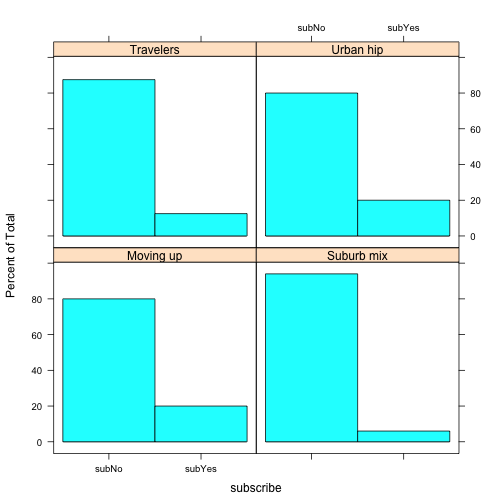### Histograms continued

You can plot counts instead of proportions with type=“count”. There are options for the layout (cols, rows in this case) and colors:

``````histogram(~subscribe | Segment, data=seg.df, type="count",
layout=c(4,1), col=c("burlywood", "darkolivegreen"))
``````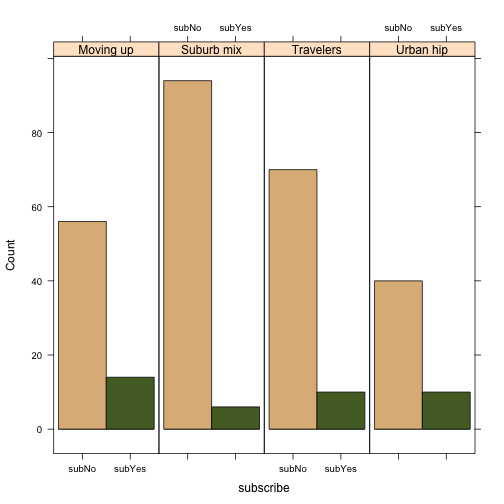# counts instead of proportions, and some visual options

### Histograms by 2 factors

Break out by multiple factors using | var1 + var2 + …:

``````histogram(~subscribe | Segment + ownHome, data=seg.df)
``````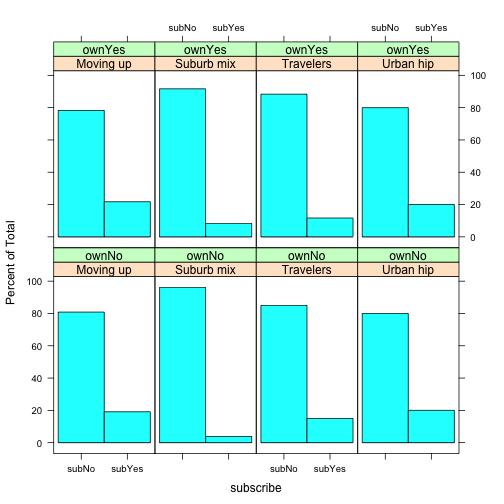The general process is to aggregate() the data that you want, then plot that. For example: mean income by segment, using a barchart:

``````seg.mean <- aggregate(income ~ Segment, data=seg.df, mean)
library(lattice)
barchart(income ~ Segment, data=seg.mean, col="grey")
``````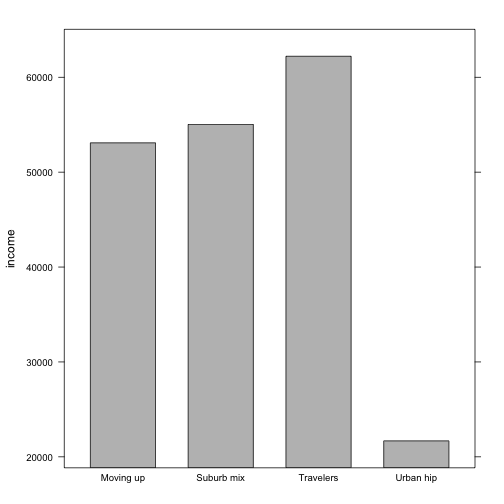### Continuous data by two factors

Use aggregate with + to break out multiple factors:

``````seg.agg <- aggregate(income ~ Segment + ownHome, data=seg.df, mean)
barchart(income ~ Segment, data=seg.agg,
groups=ownHome, auto.key=TRUE,
par.settings = simpleTheme(col=c("gray95", "gray50")) )
``````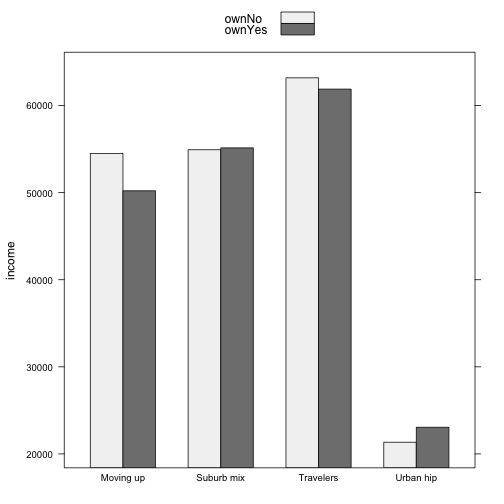### Continuous Data: "Statistics" style

Boxplots show much more information about the data distribution (see book for details). **`bwplot()`** from **`lattice`** is an upgrade over `boxplot()` that we saw in earlier chapters:

``````library(lattice)
bwplot(Segment ~ income, data=seg.df, horizontal=TRUE,
xlab = "Income")
``````